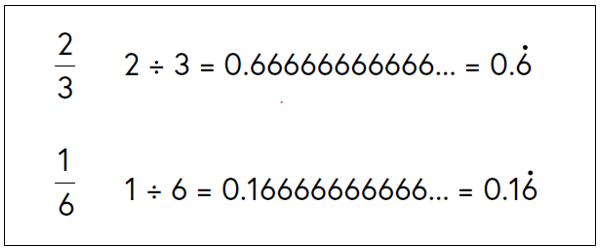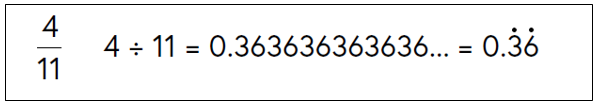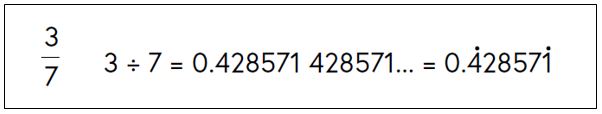## Want to keep learning?

This content is taken from the National STEM Learning Centre's online course, Maths Subject Knowledge: Fractions, Decimals, and Percentages. Join the course to learn more.
2.4

# Terminating and recurring decimals

There are three types of decimal numbers:

• Terminating decimals: these have a finite number of digits after the decimal point.
• Recurring decimals: these have one or more repeating numbers or sequences of numbers after the decimal point, which continue infinitely.
• Decimals which go on for ever, never ending and never forming a repeating pattern. These numbers are called irrational numbers and cannot be expressed as a fraction. We cover these decimals in the number course.

In this section we explore terminating and repeating decimals.

## Example: terminating decimal

The fraction $$\frac{3}{5}$$ when expressed as a decimal equals 0.6 and the fraction $$\frac{4}{25}$$ when expressed as decimal is 0.16.

The fractions $$\frac{3}{5}$$ and $$\frac{4}{25}$$ can be expressed as terminating decimals. These are numbers with a finite number of decimal places.

## Example: recurring decimal

The fraction $$\frac{2}{3}$$ when expressed as a decimal produces a decimal which never ends: a recurring decimal. $$\frac{2}{3}$$ when expressed as a decimal is 0.666666666666… $$\frac{1}{6}$$ when expressed as a decimal is 0.166666666666…

### Writing recurring decimals

We write recurring decimal using a dot over the recurring digit like this.Sometimes not just one digit recurs in the decimal part. In this example of four elevenths, the 3 and the 6 recur. We place a dot over the three and the six to signify that both digits recur.A whole sequence of digits can recur. In these cases we do not place a dot over each of the recurring digits, just over the first and the last digits in the recurring sequence of digits.